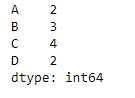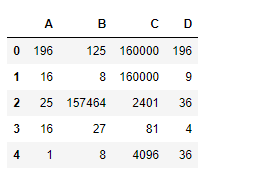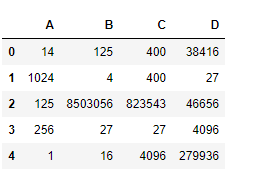# Python | Pandas dataframe.pow()

Python is a great language for doing data analysis, primarily because of the fantastic ecosystem of data-centric python packages. Pandas is one of those packages and makes importing and analyzing data much easier.

Pandas` dataframe.pow()` function calculates the exponential power of dataframe and other, element-wise (binary operator pow). This function is essentially same as the `dataframe ** other` but with a support to fill the missing values in one of the input data.

Syntax: DataFrame.pow(other, axis=’columns’, level=None, fill_value=None)

Parameters :
other : Series, DataFrame, or constant
axis : For Series input, axis to match Series index on
level : Broadcast across a level, matching Index values on the passed MultiIndex level
fill_value : Fill existing missing (NaN) values, and any new element needed for successful DataFrame alignment, with this value before computation. If data in both corresponding DataFrame locations is missing the result will be missing.
**kwargs : Additional keyword arguments are passed into DataFrame.shift or Series.shift.

Returns : result : DataFrame

Example #1: Use `pow()` function to find the power of each element in the dataframe. Raise each element in a row to a different power using a series.

 `# importing pandas as pd ` `import` `pandas as pd ` ` `  `# Creating the dataframe  ` `df1 ``=` `pd.DataFrame({``"A"``:[``14``, ``4``, ``5``, ``4``, ``1``], ` `                    ``"B"``:[``5``, ``2``, ``54``, ``3``, ``2``], ` `                    ``"C"``:[``20``, ``20``, ``7``, ``3``, ``8``], ` `                    ``"D"``:[``14``, ``3``, ``6``, ``2``, ``6``]}) ` ` `  `# Print the dataframe ` `df `Let’s create a Series

 `# importing pandas as pd ` `import` `pandas as pd ` ` `  `# Create the Series ` `sr ``=` `pd.Series([``2``, ``3``, ``4``, ``2``], index ``=``[``"A"``, ``"B"``, ``"C"``, ``"D"``]) ` ` `  `# Print the series ` `sr `Now, let’s use the `dataframe.pow()` function to raise each element in a row to different power.

 `# find the power ` `df.``pow``(sr, axis ``=` `1``) `

Output :Example #2: Use `pow()` function to raise each element of first data frame to the power of corresponding element in the other dataframe.

 `# importing pandas as pd ` `import` `pandas as pd ` ` `  `# Creating the first dataframe  ` `df1 ``=` `pd.DataFrame({``"A"``:[``14``, ``4``, ``5``, ``4``, ``1``], ` `                    ``"B"``:[``5``, ``2``, ``54``, ``3``, ``2``],  ` `                    ``"C"``:[``20``, ``20``, ``7``, ``3``, ``8``], ` `                    ``"D"``:[``14``, ``3``, ``6``, ``2``, ``6``]}) ` ` `  `# Creating the second dataframe ` `df2 ``=` `pd.DataFrame({``"A"``:[``1``, ``5``, ``3``, ``4``, ``2``], ` `                    ``"B"``:[``3``, ``2``, ``4``, ``3``, ``4``], ` `                    ``"C"``:[``2``, ``2``, ``7``, ``3``, ``4``], ` `                    ``"D"``:[``4``, ``3``, ``6``, ``12``, ``7``]}) ` ` `  `# using pow() function to raise each element ` `# in df1 to the power of corresponding element in df2 ` `df1.``pow``(df2) `

Output :My Personal Notes arrow_drop_upCheck out this Author's contributed articles.

If you like GeeksforGeeks and would like to contribute, you can also write an article using contribute.geeksforgeeks.org or mail your article to contribute@geeksforgeeks.org. See your article appearing on the GeeksforGeeks main page and help other Geeks.

Please Improve this article if you find anything incorrect by clicking on the "Improve Article" button below.

Article Tags :

Be the First to upvote.

Please write to us at contribute@geeksforgeeks.org to report any issue with the above content.